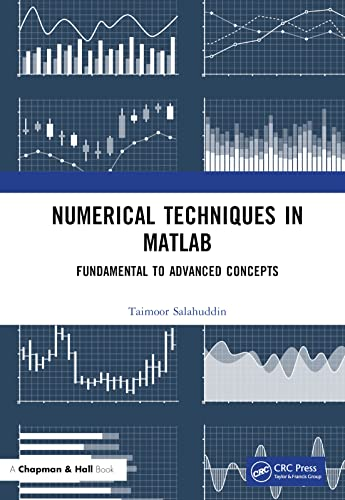# Numerical Techniques in MATLAB: Fundamental to Advanced Concepts

• Length: 216 pages
• Edition: 1
• Publisher:
• Publication Date: 2023-09-27
• ISBN-10: 1032472588
• ISBN-13: 9781032472584
Description

In this book, various numerical methods are discussed in a comprehensive way. It delivers a mixture of theory, examples and MATLAB® practicing exercises to help the students in improving their skills. To understand the MATLAB programming in a friendly style, the examples are solved. The MATLAB codes are mentioned in the end of each topic. Throughout the text, a balance between theory, examples and programming is maintained.

Key Features

Methods are explained with examples and codes
System of equations has given full consideration
Use of MATLAB is learnt for every method

This book is suitable for graduate students in mathematics, computer science and engineering.